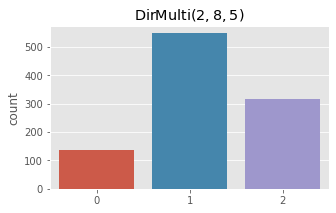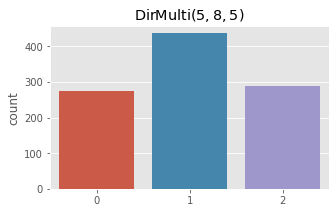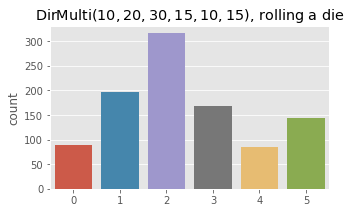# 3. Dirichlet-Multinomial Distribution

This notebook is about the Dirichlet-Multinomial distribution. This distribution has a wide ranging array of applications to modelling categorical variables. It has found its way into machine learning areas such as topic modeling and Bayesian Belief networks. The Dirichlet-Multinomial probability mass function is defined as follows.

$$\Pr(\mathbf{x}\mid\boldsymbol{\alpha})=\frac{\left(n!\right)\Gamma\left(\alpha_0\right)} {\Gamma\left(n+\alpha_0\right)}\prod_{k=1}^K\frac{\Gamma(x_{k}+\alpha_{k})}{\left(x_{k}!\right)\Gamma(\alpha_{k})}$$

where

• $$x_k$$ is the k-th count

• $$n = \sum_k x_k$$

• $$\alpha_k$$ is a prior belief about the k-th count

• $$\alpha_0 = \sum_k \alpha_k$$

• $$\Gamma$$ is the gamma function, defined as $$\Gamma(x) = (x -1)!$$

To make this distribution easier to understand, let’s imagine an unfair die (die is the singular form of dice). Let’s remember that a die has 6 sides. Now, with this 6-sided die, we roll it 100 times and observe the number of times a 1, 2, 3, 4, 5, and 6 came up.

• 1: 10

• 2: 20

• 3: 30

• 4: 15

• 5: 10

• 6: 15

We can model the probability distribution of these numbers using a Multinomial distribution, which is defined as follows.

$$f(x_1,\ldots,x_k;p_1,\ldots,p_k) = \frac{n!}{x_1!\cdots x_k!} p_1^{x_1} \cdots p_k^{x_k}$$

where

• $$x_1$$ is the k-th count

• $$n = \sum_k x_k$$

• $$p_k$$ is the k-th probability corresonding to the k-th count

Note the factorials? We can re-express the Multinomial distribution using the $$\Gamma$$ function.

$$f(x_1,\dots, x_{k}; p_1,\ldots, p_k) = \frac{\Gamma(\sum_i x_i + 1)}{\prod_i \Gamma(x_i+1)} \prod_{i=1}^k p_i^{x_i}$$

But how does the Dirichlet distribution fit it? The Dirichlet distribution is used with the Multinomial when we consider a Bayesian approach to modeling the distribution. In simple words, the probabilities $$p_1, \ldots, p_k$$ are the parameters of the counts $$x_1, \ldots, x_k$$, and the priors $$\alpha_1, \ldots, \alpha_k$$ are the parameters of the probabilities. The priors $$\alpha$$ are called the hyperparameters (parameters of other parameters), and probabilities are called the parameters. The counts are generated by a multinomial distribution, and the multinomial distribution probabilities $$p_k$$’s are generated by a Dirichlet distribution.

The Dirichlet distribution is defined as follows.

$$f \left(p_1,\ldots, p_{K}; \alpha_1,\ldots, \alpha_K \right) = \frac{1}{\mathrm{B}(\boldsymbol\alpha)} \prod_{i=1}^K p_i^{\alpha_i - 1}$$

where

• $$\mathrm{B}(\boldsymbol\alpha) = \frac{\prod_{i=1}^K \Gamma(\alpha_i)}{\Gamma\left(\sum_{i=1}^K \alpha_i\right)}$$

Just trust me that you can combine the Dirichlet with the Multinomial to get the final expression below.

$$f(x_1,\ldots,x_k;\alpha_1,\ldots,\alpha_k) = \frac{\left(n!\right)\Gamma\left(\alpha_0\right)}{\Gamma\left(n+\alpha_0\right)}\prod_{k=1}^K\frac{\Gamma(x_{k}+\alpha_{k})}{\left(x_{k}!\right)\Gamma(\alpha_{k})}$$

This notebook shows how you can code up all the distributions and functions involved with Dirichlet-Multinomial distribution. It also shows how you can use the distribution to compute the log-probability of the data as well as sample data from the distribution.

## 3.1. Functions and distributions

Below are the code to express the functions and distributions.

• The factorial function computes $$x!$$. However, for large $$x$$, the evaluation will lead to overflow, and so we use Stirling’s approximation.

• The gamma function $$\Gamma(x)$$ uses the factorial function.

• The beta function $$\mathrm{B}(\alpha_1, \ldots, \alpha_K)$$ uses the gamma function.

• The gamma distribution (note there is the gamma function, above, and this one, the gamma distribution) $$\Gamma(x; \alpha, \beta)$$ is usde by the Dirichlet distribution. As you can see, there are 2 parameters $$\alpha$$ and $$\beta$$, but our implementation below only considers $$\alpha$$ with $$\beta$$ assumed to be 1. Also note that in general, $$\alpha$$ and $$\beta$$ can take on values other than integers; in this implementation, we only consider whole numbers (I think the program might aslpode if you put in floats).

• The Dirichlet-Multinomial distribution uses the Dirichlet and Multinomial distributions.

:

%matplotlib inline
import numpy as np
import random
import seaborn as sns
import matplotlib.pylab as plt

random.seed(37)
np.random.seed(37)
plt.style.use('ggplot')

def factorial(x):
if x <= 0.0:
return 1

# typically stirling's approximation would be (x / e)^x
# for large values of x, this creates an overflow
# we use 1 here, which doesn't seem to affect the sampling or probability estimations
g = np.sqrt(2 * np.pi * x) * np.power(x / 2.718281, 1)
return g

def gamma(n):
return factorial(n - 1)

def beta(alphas):
k = len(alphas)
b = 1.
for i in range(k):
b *= gamma(alphas[i])
b = b / gamma(np.sum(alphas))
return b

def rgama(a):
d = a - 1. / 3.
c = 1. / np.sqrt(9. * d)

while True:
x = None
v = -1

while v <= 0:
x = np.random.normal(0, 1)
v = 1. + c * x

v = np.power(v, 3)
u = np.random.uniform()

if u < 1 - 0.0331 * (x * x) * (x * x):
return d * v

if np.log(u) < 0.5 * x * x + d * (1 - v + np.log(v)):
return d * v

def dgama(x, alpha):
d = np.log(np.power(x, alpha - 1)) + np.log(2.718281) - np.log(gamma(alpha))
return d

def rdirch(alphas):
k = len(alphas)
x = np.array([rgama(alphas[i]) for i in range(k)])
total = np.sum(x)
x = [s / total for s in x]
return x

def ddirch(x, alphas):
k = len(x)
d = 0.
for i in range(k):
d += np.log(np.power(x[i], alphas[i] - 1))
d -= np.log(1. / beta(alphas))
return d

def rmultnomial(p):
k = len(p)
probs = [prob / np.sum(p) for prob in p]
x = np.random.uniform()
cummulative_p = 0
for i, prob in enumerate(probs):
cummulative_p += prob
if cummulative_p - x >= 0:
return i
return k - 1

def dmultinomial(x, p):
k = len(x)
d = np.log(gamma(np.sum(x + 1)))
for i in range(k):
d += np.log(np.power(p[i], x[i]))
for i in range(k):
d -= np.log(gamma(x[i] + 1))
return d

def rdirmultinom(alphas):
p = rdirch(alphas)
m = rmultnomial(p)
return m

def ddirmultinom(x, alphas):
k = len(x)
n = np.sum(x)
sum_alphas = np.sum(alphas)

d = np.log(factorial(n))
d += np.log(gamma(sum_alphas))
d -= np.log(gamma(n + sum_alphas))

for i in range(k):
d += np.log(gamma(x[i] + alphas[i]))
d -= np.log(factorial(x[i]))
d -= np.log(gamma(alphas[i]))

return d


## 3.2. Gamma

Here, we sample 1 point from the $$\Gamma(\alpha=1)$$ distribution. Then we evaluate the probability of that sample point with $$\Gamma$$ distributions parameterized differently. As you can see, $$P(x | \Gamma(\alpha=1))$$ is the highest (log-probability).

:

import pandas as pd

x = rgama(1)

print(f'estimating probability of {x:.5f}')

pd.DataFrame(
[(alpha + 1, dgama(x, alpha + 1)) for alpha in range(5)],
columns=['alpha', 'probability'])

estimating probability of 0.62318

:

alpha probability
0 1 1.000000
1 2 0.608139
2 3 -0.904504
3 4 -1.985623
4 5 -2.890068

Here we make 1,000 samples from gamma distributions parameterized differently and plot the density curves.

:

fig, ax = plt.subplots(1, 1, figsize=(10, 5))
ax.set_xlim([0, 10])
ax.set_title(r'$\Gamma(\alpha, \beta)$')

_ = sns.kdeplot([rgama(1) for _ in range(1000)], bw=0.5, ax=ax, label=r'$\Gamma(1, 1)$')
_ = sns.kdeplot([rgama(2) for _ in range(1000)], bw=0.5, ax=ax, label=r'$\Gamma(2, 1)$')
_ = sns.kdeplot([rgama(3) for _ in range(1000)], bw=0.5, ax=ax, label=r'$\Gamma(3, 1)$')
_ = sns.kdeplot([rgama(4) for _ in range(1000)], bw=0.5, ax=ax, label=r'$\Gamma(4, 1)$')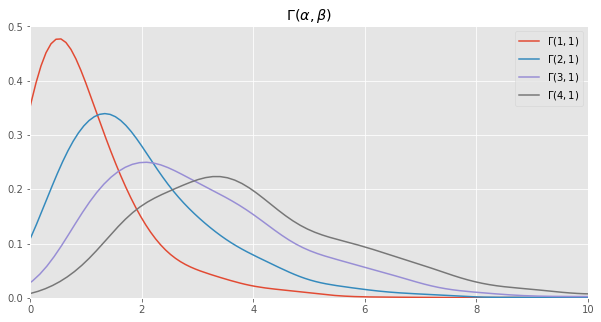## 3.3. Dirichlet

Here we sample 1 set of probabilities from a Dirichlet distribution with the $$\alpha$$’s as $$\mathrm{\alpha} = [\alpha_1, \alpha_2] = [1, 2]$$. We then estimate the (log) probability of these probabilities for two Dirichlet distribution parameterized differently.

:

alphas = np.array([1, 2])
x = rdirch(alphas)

params = [
np.array([1, 2]),
np.array([1, 3]),
np.array([5, 5]),
np.array([5, 1])
]
print(f'estimating the probability of {x}')

pd.DataFrame([(p, ddirch(x, p)) for p in params], columns=['alphas', 'probability'])

estimating the probability of [0.8011123776705179, 0.198887622329482]

:

alphas probability
0 [1, 2] -2.654736
1 [1, 3] -3.838228
2 [5, 5] -6.565092
3 [5, 1] -1.221732

Here we sample data points from two Dirichlet distributions and plot the density of the samples.

• $$\operatorname{Dir}(\alpha_1=1, \alpha_2=2)$$

• $$\operatorname{Dir}(\alpha_1=9, \alpha_2=1)$$

:

fig, ax = plt.subplots(1, 1, figsize=(10, 5))
ax.set_xlim([0, 1])
ax.set_title(r'$\operatorname{Dir}(1, 2)$')
x = np.array([rdirch(np.array([1, 2])) for _ in range(1000)])
_ = sns.distplot(x[:, 0], ax=ax, label=r'$\alpha_1$')
_ = sns.distplot(x[:, 1], ax=ax, label=r'$\alpha_2$')
_ = plt.legend()

fig, ax = plt.subplots(1, 1, figsize=(10, 5))
ax.set_xlim([0, 1])
ax.set_title(r'$\operatorname{Dir}(9, 1)$')
x = np.array([rdirch(np.array([9, 1])) for _ in range(1000)])
_ = sns.distplot(x[:, 0], ax=ax, label=r'$\alpha_1$')
_ = sns.distplot(x[:, 1], ax=ax, label=r'$\alpha_2$')
_ = plt.legend()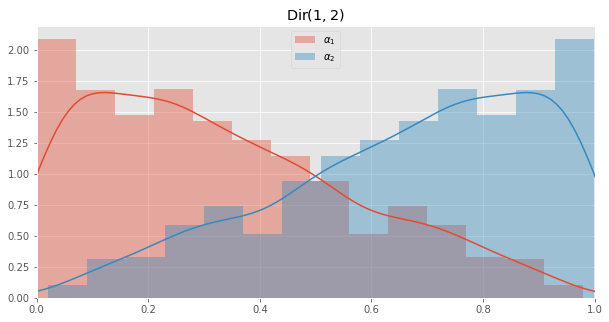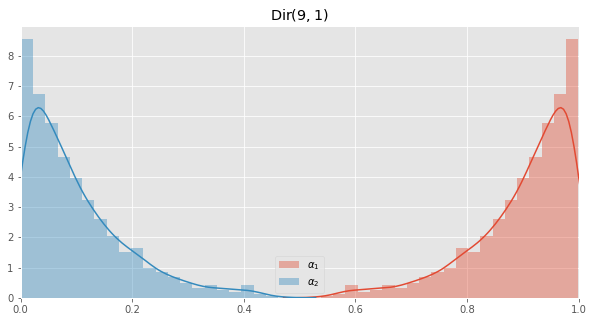## 3.4. Multinomial

Here we sample from the Multinomial distribution using different parameters. One of these sampling is for the die example at the beggining.

:

num_ones = 0
for i in range(1000):
v = rmultnomial(np.array([0.1, 0.9]))
if v == 1:
num_ones += 1

print('k = 2, p = [0.1, 0.9]')
print(num_ones / 1000.)

num_zeros = 0
num_ones = 0
num_twos = 0

for i in range(1000):
v = rmultnomial(np.array([0.1, 0.8, 0.1]))
if v == 0:
num_zeros += 1
elif v == 1:
num_ones += 1
else:
num_twos += 1

print('')
print('k = 3, p = [0.1, 0.8, 0.1]')
print(num_zeros / 1000.)
print(num_ones / 1000.)
print(num_twos / 1000.)

die_counts = np.zeros(6)
for i in range(1000):
v = rmultnomial(np.array([0.10, 0.2, 0.3, 0.15, 0.10, 0.15]))
die_counts[v] += 1
sum_counts = np.sum(die_counts)
die_counts = [c / sum_counts for c in die_counts]
print('')
print('k = 6, p = [0.1, 0.2, 0.3, 0.15, 0.10, 0.15], rolling a die')
for i, p in enumerate(die_counts):
print('{}: {}'.format(i+1, p))

k = 2, p = [0.1, 0.9]
0.895

k = 3, p = [0.1, 0.8, 0.1]
0.106
0.793
0.101

k = 6, p = [0.1, 0.2, 0.3, 0.15, 0.10, 0.15], rolling a die
1: 0.083
2: 0.197
3: 0.31
4: 0.147
5: 0.079
6: 0.184

:

fig, ax = plt.subplots(1, 1, figsize=(5, 3))
ax.set_title(r'$\operatorname{Multi}(0.1, 0.9)$')
x = np.array([rmultnomial(np.array([0.1, 0.9])) for _ in range(1000)])
_ = sns.countplot(x)

fig, ax = plt.subplots(1, 1, figsize=(5, 3))
ax.set_title(r'$\operatorname{Multi}(0.65, 0.35)$')
x = np.array([rmultnomial(np.array([0.65, 0.35])) for _ in range(1000)])
_ = sns.countplot(x)

fig, ax = plt.subplots(1, 1, figsize=(5, 3))
ax.set_title(r'$\operatorname{Multi}(0.10, 0.2, 0.3, 0.15, 0.10, 0.15)$, die rolls')
x = np.array([rmultnomial(np.array([0.10, 0.2, 0.3, 0.15, 0.10, 0.15])) for _ in range(1000)])
_ = sns.countplot(x)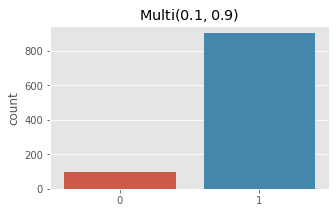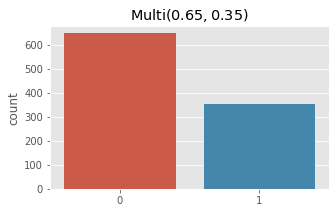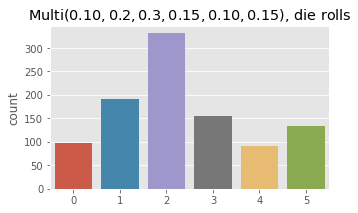Now we estimate log probability of a sample for Multinomial distributions parameterized differently.

:

import warnings

with warnings.catch_warnings(record=True):
sample = np.array([2, 8])
params = [np.array([p, 1.0 - p]) for p in np.linspace(0, 1, 11)]
sparams = ['[' + ', '.join(['{:.3f}'.format(i) for i in p]) + ']' for p in params]

df = pd.DataFrame([(s, dmultinomial(sample, p)) for p, s in zip(params, sparams)],
columns=['parameters', 'probability'])
df

:

parameters probability
0 [0.000, 1.000] -inf
1 [0.100, 0.900] -5.929033
2 [0.200, 0.800] -5.485003
3 [0.300, 0.700] -5.742324
4 [0.400, 0.600] -6.400165
5 [0.500, 0.500] -7.412451
6 [0.600, 0.400] -8.832956
7 [0.700, 0.300] -10.826111
8 [0.800, 0.200] -13.802769
9 [0.900, 0.100] -19.112381
10 [1.000, 0.000] -inf

## 3.5. Dirichlet-multinomial

Here’s the last example of sampling from a Dirichlet-Multinomial distribution and then estimating the probality of observed counts using the Dirichlet-Multinomial distributions parameterized differently.

:

count_0 = 0
count_1 = 0

for i in range(100):
v = rdirmultinom(np.array([2, 8]))
if v == 0:
count_0 += 1
else:
count_1 += 1

print('estimating probability of count_0 = {}, count_1 = {}'.format(count_0, count_1))

sample = np.array([count_0, count_1])
params = [np.array([alpha_0, 10.0 - alpha_0]) for alpha_0 in np.linspace(0, 10, 11)]

pd.DataFrame([(p, ddirmultinom(sample, p)) for p in params],
columns=['alphas', 'probability'])

estimating probability of count_0 = 17, count_1 = 83

:

alphas probability
0 [0.0, 10.0] -0.065782
1 [1.0, 9.0] 0.185436
2 [2.0, 8.0] 0.535957
3 [3.0, 7.0] -0.208196
4 [4.0, 6.0] -0.482921
5 [5.0, 5.0] -0.523687
6 [6.0, 4.0] -0.374440
7 [7.0, 3.0] 0.009409
8 [8.0, 2.0] 0.863991
9 [9.0, 1.0] 0.625902
10 [10.0, 0.0] 0.489876

Finally, we sample from a Dirichlet-Multinomial distribution and plot the observed counts.

:

fig, ax = plt.subplots(1, 1, figsize=(5, 3))
ax.set_title(r'$\operatorname{DirMulti}(2, 8, 5)$')
x = np.array([rdirmultinom(np.array([2, 8, 5])) for _ in range(1000)])
_ = sns.countplot(x)

fig, ax = plt.subplots(1, 1, figsize=(5, 3))
ax.set_title(r'$\operatorname{DirMulti}(5, 8, 5)$')
x = np.array([rdirmultinom(np.array([5, 8, 5])) for _ in range(1000)])
_ = sns.countplot(x)

fig, ax = plt.subplots(1, 1, figsize=(5, 3))
ax.set_title(r'$\operatorname{DirMulti}(10, 20, 30, 15, 10, 15)$, rolling a die')
x = np.array([rdirmultinom(np.array([10, 20, 30, 15, 10, 15])) for _ in range(1000)])
_ = sns.countplot(x)